upm  1.7.1 Sensor/Actuator repository for libmraa (v2.0.0)
LSM303D Class Reference

API for the LSM303D 3-Axis Geomagnetic Sensor. More...

## Detailed Description

The LSM303D is an ultra-low-power high-performance system-in-package featuring a 3D digital linear acceleration sensor and a 3D digital magnetic sensor. The LSM303D has linear acceleration full scales of 2g/4g/8g/16g and a magnetic field dynamic range of 50 Gauss.

Not all functionality of this chip has been implemented in this driver, however all the pieces are present to add any desired functionality. This driver supports only I2C operation.

This device requires 3.3v operation.

// Instantiate an LSM303D using default I2C parameters
upm::LSM303D sensor;
// now output data every 250 milliseconds
while (shouldRun) {
float x, y, z;
sensor.update();
sensor.getAccelerometer(&x, &y, &z);
cout << "Accelerometer x: " << x << " y: " << y << " z: " << z << " g" << endl;
sensor.getMagnetometer(&x, &y, &z);
cout << "Magnetometer x: " << x << " y: " << y << " z: " << z << " uT" << endl;
cout << "Temperature: " << sensor.getTemperature() << " C" << endl;
cout << endl;
upm_delay_us(250000);
}

## Public Member Functions

~LSM303D ()

void update ()

void getMagnetometer (float *x, float *y, float *z)

std::vector< float > getMagnetometer ()

void getAccelerometer (float *x, float *y, float *z)

std::vector< float > getAccelerometer ()

float getTemperature ()

void init (LSM303D_M_RES_T res)

void setAccelerometerFullScale (LSM303D_AFS_T fs)

void setMagnetometerFullScale (LSM303D_MFS_T fs)

void setAccelerometerODR (LSM303D_AODR_T odr)

void setMagnetometerODR (LSM303D_MODR_T odr)

## Protected Member Functions

uint8_t readReg (uint8_t reg)

int readRegs (uint8_t reg, uint8_t *buffer, int len)

void writeReg (uint8_t reg, uint8_t val)

## Protected Attributes

lsm303d_context m_lsm303d

## Constructor & Destructor Documentation

 LSM303D ( int bus = `LSM303D_DEFAULT_I2C_BUS`, int addr = `LSM303D_DEFAULT_I2C_ADDR` )

LSM303D constructor

This driver only supports I2C.

Due to the fact that this chip is currently obsolete, we only support minimum functionality.

Parameters
 bus I2C bus to use addr The I2C address of the device
Returns
The device context, or NULL if an error occurred
Exceptions
 std::runtime_error on failure.
 ~LSM303D ( )

LSM303D Destructor

Here is the call graph for this function: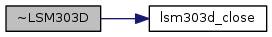## Member Function Documentation

 void update ( void )

Update the internal stored values from sensor data. This method must be called before querying the acceleration, magnetometer, or temperature.

Exceptions
 std::runtime_error on failure

Here is the call graph for this function: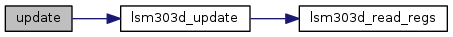void getMagnetometer ( float * x, float * y, float * z )

Return magnetometer data in micro-Teslas (uT). update() must have been called prior to calling this method.

Parameters
 x Pointer to a floating point value that will have the current x component placed into it y Pointer to a floating point value that will have the current y component placed into it z Pointer to a floating point value that will have the current z component placed into it

Here is the call graph for this function: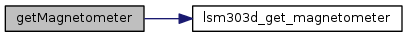std::vector< float > getMagnetometer ( )

Return magnetometer data in micro-Teslas (uT) in the form of a floating point vector. update() must have been called prior to calling this method.

Returns
A floating point vector containing x, y, and z in that order
 void getAccelerometer ( float * x, float * y, float * z )

Return acceleration data in gravities. update() must have been called prior to calling this method.

Parameters
 x Pointer to a floating point value that will have the current x component placed into it y Pointer to a floating point value that will have the current y component placed into it z Pointer to a floating point value that will have the current z component placed into it

Here is the call graph for this function: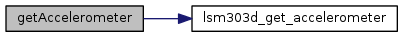std::vector< float > getAccelerometer ( )

Return acceleration data in gravities in the form of a floating point vector. update() must have been called prior to calling this method.

Returns
A floating point vector containing x, y, and z in that order
 float getTemperature ( void )

Return temperature data in degrees Celsius. NOTE: This is not the ambient room temperature. update() must have been called prior to calling this method.

Returns
Temperature in degrees Celsius

Here is the call graph for this function: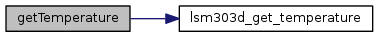void init ( LSM303D_M_RES_T res )

Initialize the device and start operation. This function is called from the constructor so it will not typically need to be called by a user unless the device is reset. It will initialize the accelerometer and magnetometer (if enabled) to certain default running modes.

Parameters
 res One of the LSM303D_M_RES_T values. This value sets the resolution of the magnetometer. At init time, this value is set to LSM303D_M_RES_HIGH.
Exceptions
 std::runtime_error on failure

Here is the call graph for this function: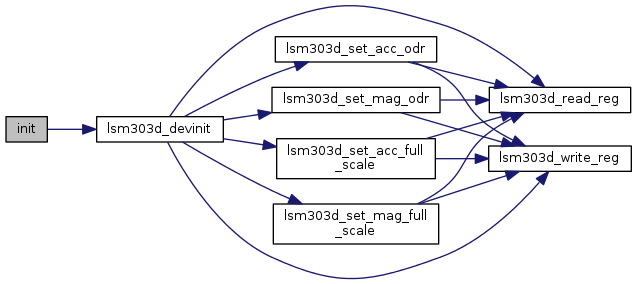void setAccelerometerFullScale ( LSM303D_AFS_T fs )

Set the full scale (sensitivity) value for the accelerometer. This device supports 2G, 4G, 6G, 8G, and 16G full scale modes.

Parameters
 fs One of the LSM303D_AFS_T values
Exceptions
 std::runtime_error on failure

Here is the call graph for this function:void setMagnetometerFullScale ( LSM303D_MFS_T fs )

Set the full scale (sensitivity) value for the magnetometer. This device supports 2, 4, 8, and 16 Gauss full scale modes.

Parameters
 fs One of the LSM303D_MFS_T values
Exceptions
 std::runtime_error on failure

Here is the call graph for this function: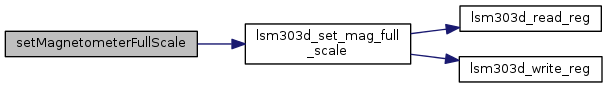void setAccelerometerODR ( LSM303D_AODR_T odr )

Set the accelerometer output data rate (ODR)

Parameters
 odr One of the LSM303D_AODR_T values. The default set at initialization time is LSM303D_AODR_100HZ.
Exceptions
 std::runtime_error on failure

Here is the call graph for this function:void setMagnetometerODR ( LSM303D_MODR_T odr )

Set the magnetometer output data rate (ODR)

Parameters
 odr One of the LSM303D_MODR_T values. The default set at initialization time is LSM303D_MODR_12_5HZ.
Exceptions
 std::runtime_error on failure

Here is the call graph for this function: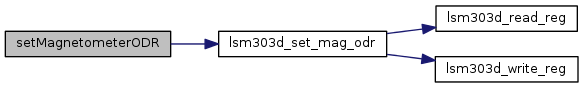uint8_t readReg ( uint8_t reg )
protected

Parameters
 reg The register to read
Returns
The value of the register

Here is the call graph for this function: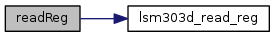int readRegs ( uint8_t reg, uint8_t * buffer, int len )
protected

Read contiguous registers into a buffer

Parameters
 buffer The buffer to store the results len The number of registers to read
Returns
The number of bytes read
Exceptions
 std::runtime_error on failure

Here is the call graph for this function: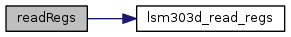void writeReg ( uint8_t reg, uint8_t val )
protected

Write to a register

Parameters
 reg The register to write to val The value to write
Exceptions
 std::runtime_error on failure

Here is the call graph for this function: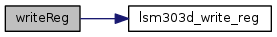Collaboration diagram for LSM303D: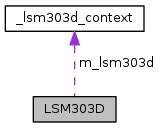[legend]

The documentation for this class was generated from the following files: